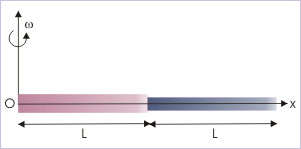# 20.6 Conservation of angular momentum (application)  (Page 4/6)

 Page 4 / 6The rod slips away from the axis of rotation.

We may question why the same does not happen with a particle of rigid body in rotation. We know that rigid body does not allow relative displacement of particles. The particles are in place due to intermolecular forces operating on them. The tendency of the particle to move away from the axis of rotation is counteracted by the net inter-molecular force on the particle that acts towards the center of rotation. In this case, the inter-molecular force meets the requirement of centripetal force for circular motion of the particle about the axis of rotation.

Now, we must check about external torque on the system. The system lies on the smooth horizontal plane. It means that there is no net vertical force (weights of the bodies are balanced by normal forces). The force at the axis does not constitute torque as its moment arm is zero. We, therefore, conclude that there is no external torque on the system and that the problem can be analyzed in terms of conservation of angular momentum.

In the beginning, when the system is given initial angular velocity, the system has certain angular momentum, “ ${L}_{i}$ ”. During the motion, however, rod begins to slip away from the axis. As such, mass distribution of the system changes. In other words, MI of the system changes. But, the angular momentum of the system (" ${L}_{f}$ ") remains unchanged as there is no external torque on the system.

Now, applying conservation of angular momentum in vertical direction :

$\begin{array}{l}{L}_{i}={L}_{f}\\ {I}_{i}{\omega }_{i}={I}_{f}{\omega }_{f}\end{array}$

Here, ${\omega }_{i}=\omega$ . According to theorem of parallel axes, the initial MI of the system, “ ${I}_{i}$ ”, is :

$\begin{array}{l}{I}_{i}=\phantom{\rule{2pt}{0ex}}\text{MI of the system about perpendicular at mid-point}\phantom{\rule{4pt}{0ex}}\\ \phantom{\rule{4pt}{0ex}}+\phantom{\rule{4pt}{0ex}}\text{mass of the system x square of perpendicular distance between the axes}\end{array}$

$\begin{array}{l}{I}_{i}=\left\{\left(\frac{2M{L}^{2}}{12}+2M{\left(\frac{L}{2}\right)}^{2}\right\}\end{array}$

$\begin{array}{l}⇒{I}_{i}=\frac{M{L}^{2}}{6}+\frac{M{L}^{2}}{2}=\frac{2M{L}^{2}}{3}\end{array}$

Note that we have used "2M" to account for both tube and rod. Now, we again employ theorem of parallel axes in order to obtain MI of the system for the final position, when the rod just slips out of the tube. Final MI of the system, “ ${I}_{f}$ ”, is :The rod slips away from the axis of rotation.

$\begin{array}{l}{I}_{f}=\left\{\left(\frac{M{L}^{2}}{12}+M{\left(\frac{L}{2}\right)}^{2}+\frac{M{L}^{2}}{12}+M{\left(\frac{3L}{2}\right)}^{2}\right\}\end{array}$

$\begin{array}{l}⇒{I}_{f}=\frac{M{L}^{2}}{6}+\frac{M{L}^{2}}{4}+\frac{9M{L}^{2}}{4}\end{array}$

$\begin{array}{l}⇒{I}_{f}=\frac{2M{L}^{2}+3M{L}^{2}+27M{L}^{2}}{12}=\frac{8M{L}^{2}}{3}\end{array}$

Putting values in the equation of conservation of angular momentum, we have :

$\begin{array}{l}⇒\frac{2M{L}^{2}}{3}x\omega =\frac{8M{L}^{2}}{3}x{\omega }_{f}\end{array}$

$\begin{array}{l}⇒{\omega }_{f}=\frac{\omega }{4}\end{array}$

QBA (Question based on above) : A thin rod is placed coaxially within a thin hollow tube, which lies on a smooth horizontal table. The rod and tube are of same mass “M” and length “L”. The rod is free to move within the tube. The system of tube and rod is given an initial angular velocity, “ω”, about a vertical axis passing through the center of the system. Considering negligible friction between surfaces, find the angular velocity of the rod, when it just slips out of the tube.

Hint : The question differs in one respect to earlier question. The axis of rotation here is vertical axis through the center of the system instead of the axis at the end of the system. This changes the calculation of MIs before and after. Answer is “ω/7”.

#### Questions & Answers

List the application of projectile
Luther Reply
How can we take advantage of our knowledge about motion?
Kenneth Reply
pls explain what is dimension of 1in length and -1 in time ,what's is there difference between them
Mercy Reply
what are scalars
Abdhool Reply
show that 1w= 10^7ergs^-1
Lawrence Reply
what's lamin's theorems and it's mathematics representative
Yusuf Reply
if the wavelength is double,what is the frequency of the wave
Ekanem Reply
What are the system of units
Jonah Reply
A stone propelled from a catapult with a speed of 50ms-1 attains a height of 100m. Calculate the time of flight, calculate the angle of projection, calculate the range attained
Samson Reply
58asagravitasnal firce
Amar
water boil at 100 and why
isaac Reply
what is upper limit of speed
Riya Reply
what temperature is 0 k
Riya
0k is the lower limit of the themordynamic scale which is equalt to -273 In celcius scale
Mustapha
How MKS system is the subset of SI system?
Clash Reply
which colour has the shortest wavelength in the white light spectrum
Mustapha Reply
how do we add
Jennifer Reply
if x=a-b, a=5.8cm b=3.22 cm find percentage error in x
Abhyanshu Reply
x=5.8-3.22 x=2.58
sajjad

### Read also:

#### Get Jobilize Job Search Mobile App in your pocket Now!

Source:  OpenStax, Physics for k-12. OpenStax CNX. Sep 07, 2009 Download for free at http://cnx.org/content/col10322/1.175
Google Play and the Google Play logo are trademarks of Google Inc.

Notification Switch

Would you like to follow the 'Physics for k-12' conversation and receive update notifications?By Cath YuBy Hope PercleBy Jazzycazz JacksonBy Stephen VoronBy Brianna BeckBy Janet ForresterBy OpenStaxBy Saylor FoundationBy OpenStaxBy Rohini Ajay